All Maths Formulas for Class 11

# All Maths Formulas for Class 11

## All Formulas for Maths Class 11

If you are searching for all the formulas of Maths Class 11 at one place, then you are surely come to the right place. Maths-formula.com brings you all the important concepts, theories and Maths formulas for class 11 to help you in your preparation for mathematics class 11 examination. These concepts, theories and formulas are extremely important from the examinations’ point of view.

Maths is a subject where reasoning and logic are very important. Students should have a clear understanding of the underlying theories, concepts and formulas. They must understand that what are the different formulas and what they mean actually. Only then, they will be able to crack the mathematics questions asked in the examination. Here, we are providing all the concepts and theories of those chapters also which do not have the formulas and have only theories and definitions.

## Chapter-wise All Maths Formulas for Class 11

### Maths Formulas for Class 11: Chapter 1 Sets

• Set: A set is a well-defined collection of objects.
• Representation of sets: (i) Roster form or tabular form, (ii) Rule method or set builder form.

Types of Sets:

• Empty set: A set which does not contain any element is called empty set or null set or void set. It is denoted by φ or { }.
• Singleton set: A set, containing a single element, is called a singleton set.
• Finite set: A set, which contains a definite number of elements, is called a finite set.
• Infinite set: A set, which contains an infinite number of elements, is called an infinite set.
• Equivalent sets: Two finite sets A and B are equivalent, if their cardinal numbers are the same, i.e., n(A) = n(B).
• Equal sets: Two sets A and B are said to be equal, if they have exactly the same elements.
• Subset: A set A is said to be subset of a set B, if every element of A is also an element of B. Intervals are subsets of R.
• Proper set: If A  B and A ≠ B, then A is called a proper set of B, written as A  B.
• Universal set: If all the sets under consideration are subsets of a large set U, then the set U is known as a universal set. And it is denoted by rectangle in Venn-diagram.
• Power set: A power set of a set A is the collection of all the subsets of A. It is denoted by P(A).
• Venn-diagram: A geometrical figure illustrating universal set, subsets and their operations is known as Venn-diagram.
• Union of sets: The union of two sets A and B is the set of all those elements which are either in A or in B.
• Intersection of sets: The intersection of two sets A and B is the set of all elements which are common.
• Disjoint sets: Two sets A and B are said to be disjoint, if A ∩ B = φ.
• Difference of sets: The difference of two sets A and B, i.e., set (A – B) is the set of those elements of A which do not belong to B.
• Compliment of a set: The complement of a subset A of universal set U is the set of all elements of U which are not the elements of A. The compliment of a set A is denoted by A’. And A' = U – A.
• For any two sets A and B, (A B)′ = A′ ∩ B′ and ( A ∩ B )′ = A′ B′
• If A and B are finite sets such that A ∩ B = φ, then n(A B) = n(A) + n(B).
• If A and B are finite sets such that A ∩ B ≠ φ, then n(A B) = n(A) + n(B) – n(A ∩ B).

### Maths Formulas for Class 11: Chapter 2 Relations and Functions

• Ordered pair: A pair of elements grouped together in a particular order, i.e., (a, b). Clearly, (a, b) ≠ (b, a).
• Cartesian product of two sets: The Cartesian product of two sets A and B is given by A × B = {(a, b): a A, b B}.
• In particular, R × R = {(x, y): x, y R} and R × R × R = (x, y, z): x, y, z R}
• If (a, b) = (x, y), then a = x and b = y.
• If n(A) = p and n(B) = q, then n(A × B) = pq.
• A × φ = φ
• In general, A × B ≠ B × A.
• Relation: A relation R from a set A to a set B is a subset of the Cartesian product A × B obtained by describing a relationship between the first element x and the second element y of the ordered pairs in A × B, i.e., R A × B.
• Number of Relations: Let A and B be two non-empty finite sets, containing m and n elements respectively, then the total number of relations from A to B is 2mn.
• Domain: The domain of R is the set of all first elements of the ordered pairs in a relation R. Domain R = {a: (a, b) R}.
• The image of an element x under a relation R is given by y, where (x, y) R.
• Range: The range of the relation R is the set of all second elements of the ordered pairs in a relation R. Range R = {b: (a, b) R}.
• Function: A function f from a set A to a set B is a specific type of relation for which every element x of set A has one and only one image y in set B. We write f : A → B, where f(x) = y.
• Domain and Co-domain: The set A is called the domain of function f and the set B is called the co-domain of f.
• Range: If f is a function from A to B, then each element of A corresponds to one and only one element of B, whereas every element in B need not be the image of some x in A. The subset of B containing the image of elements of A is called the range of the function. The range of f is denoted by f(A). Mathematically, we write: f(A) = {f(x): x A}.
• Image: If the element x of A corresponds to y B under the function f, then we say that y is the image of x under f and we write, f(x) = y.
• Pre-image: If f(x) = y, then x is the pre-image of y.

### Maths Formulas for Class 11: Chapter 3 Trigonometric Functions

• Measurement of an angle: The measure of an angle is the amount of rotation from the initial side to the terminal side.
• Right angle: If the rotating ray starting from its initial position to final position, describes one quarter of a circle, then we say that the measure of the angle formed is a right angle.
• If in a circle of radius r, an arc of length l subtends an angle of θ radians, then l = rθ.
• Radian measure = π/180 × Degree measure
• Degree measure = 180/π × Radian measure

Trigonometric Functions

Trigonometric Identities:

• cos2 x + sin2 x = 1
• 1 + tan2 x = sec2 x
• 1 + cot2 x = cosec2 x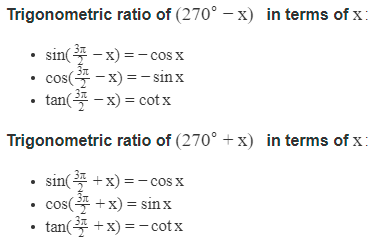Trigonometric ratio of (360 − x) in terms of x:

• cos (2π − x) = cos x
• sin (2π − x) = −sin x
• tan (2π − x) = −tan x

Trigonometric ratio of (360 + x) in terms of x:

• cos (2π + x) = cos x
• sin (2π + x) = sin x
• tan (2π + x) = tan x
• cos (2nπ + x) = cos x
• sin (2nπ + x) = sin x
• tan (2nπ + x) = tan x

Trigonometric Ratios of Compound Angles:

Sum Formulae:

Transformation Formulae
:

Product Formulae (on the basis of L.H.S.) or A-B formulae:

• 2 sin x cos y = sin (x + y) + sin (x − y)
• 2 cos x sin y = sin (x + y) – sin (x − y)
• 2 cos x cos y = cos (x + y) + cos (x − y)
• 2 sin x sin y = cos (x − y) – cos (x + y)

Sum and Difference Formulae (on the basis of L.H.S.) or C-D formulae:

Trigonometric Equations
:

• Principle Solutions: The solutions of a trigonometric equation, for which 0 ≤ x < 2π are called the principle solutions.
• General Solutions: The solution, consisting of all possible solutions of a trigonometric equation is called its general solutions.

Some General Solutions:

• sin x = 0 gives x = nπ, where n Z
• cos x = 0 gives x = (2n + 1)π/2, where n Z
• tan x = 0 gives x = nπ
• cot x = 0 gives x = (2n + 1)π/2
• sec x = 0 gives no solution
• cosec x = 0 gives no solution
• sin x = sin y gives x = nπ + (−1)ny
• cos x = cos y gives x = 2nπ ± y, where n Z
• tan x = tan y gives x = nπ + y, where n Z
• sin 2x = sin 2y gives x = nπ ± y
• cos 2x = cos 2y gives x = nπ ± y
• tan 2x = tan 2y gives x = nπ ± y

### Maths Formulas for Class 11: Chapter 4 Principle of Mathematical Induction

• One key basis for mathematical thinking is deductive reasoning. In contrast to deduction, inductive reasoning depends on working with different cases and developing a conjecture by observing incidences till we have observed each and every case. Thus, in simple language we can say the word ‘induction’ means the generalisation from particular cases or facts.
• Statement: A sentence is called a statement, if it is either true or false.
• Motivation: Motivation is tending to initiate an action. Here, basis step to motivate us for mathematical induction.
• Principle of Mathematical Induction: The principle of mathematical induction is one such tool which can be used to prove a wide variety of mathematical statements. Each such statement is assumed as P(n) associated with positive integer n, for which the correctness for the case n = 1 is examined. Then assuming the truth of P(k) for some positive integer k, the truth of P(k + 1) is established.

Working Rule:
Step 1: Show that the given statement is true for n = 1.
Step 2: Assume that the statement  is true for n = k.
Step 3: Using the assumption made in step 2, show that the statement is true for nk  + 1. We have proved the statement is true for n = k. According to step 3, it is also true for k + 1 (i.e., 1 + 1 = 2). By repeating the above logic, it is true for every natural number.

## Maths Formulas for Class 11: Chapter 5 Complex Numbers and Quadratic Equations

A number of the form a + ib, where a and b are the real numbers, is known as the complex number; a is called the real part and b is called the imaginary part of the complex number.

1. Let z1 = a + ib and z2 = c + id; then:

(i) z1 + z2 = (a + c) + i(b + d)

(ii) z1 . z2 = (acbd) – i(ad + bc)

### Maths Formulas for Class 11: Chapter 6 Linear Inequalities

·        Two real numbers or two algebraic expressions related by the symbols <, >, ≤ or ≥ form an inequality.

·        Equal numbers may be added to (or subtracted from) both sides of an inequality.

·        Both sides of an inequality can be multiplied (or divided) by the same positive number. But when both sides are multiplied (or divided) by a negative number, then the inequality is reversed.

·        The value of x, which make an inequality a true statement, are called solutions of the inequality.

·        To represent x < a (or x > a) on a number line, put a circle on the number a and dark line to the left (or right) of the number a.

·        To represent xa (or xa) on a number line, put a dark circle on the number a and dark the line to the left (or right) of the number a.

·        If an inequality is having ≤ or ≥ symbol, then the points on the line are also included in the solutions of the inequality and the graph of the inequality lies to the left (below) or right (above) of the graph of the equality represented by dark line that satisfies an arbitrary point in that part.

·        If an inequality is having < or > symbol, then the points on the line are not included in the solutions of the inequality and the graph of the inequality lies to the left (below) or right (above) of the graph of the corresponding equality represented by dotted line that satisfies an arbitrary point in that part.

·        The solution region of a system of inequalities is the region which satisfies all the given inequalities in the system simultaneously.

### Maths Formulas for Class 11: Chapter 7 Permutations and Combinations

• Addition Law: If there are two operations such that they can be performed independently in m and n ways respectively, then either of the two operations can be performed in (m + n) ways.
• Multiplication: If one operation can be performed in m ways and if corresponding to each of the m ways of performing this operation, there are n ways of performing a second operation, then the number of ways of performing two operations together in m × n.
• Factorial Notation: The continued product of first n natural numbers is called the 'n factorial' and is denoted by n!.
• 0! = 1

Permutations:

• n! = 1 × 2 × 3 × … × n! = 1 × 2 × 3 × … × n
• n! = n × (n − 1)!
• The number of permutations of n different things taken r at a time, where repetition is allowed, is given by: nr
• The number of permutations of n objects taken all at a time, where p1 objects are of first kind, p2 objects are of the second kind, …., pk objects are of kth kind and rest, if any, are different, is given by:

Combinations:

### Maths Formulas for Class 11: Chapter 8 Binomial Theorem

(a) Principle of Mathematical Induction.

(b) Combinatorial Method.

### Maths Formulas for Class 11: Chapter 9 Sequence and Series

·        An arithmetic progression (A.P.) is a sequence where the terms either increase or decrease regularly by the same constant. This constant is called the common difference (d). The first term is denoted by a and the last term of an AP is denoted by l.

·        A sequence is said to be following the rules of geometric progression or G.P. if the ratio of any term to its preceding term is specifically constant all the time. This constant factor is called the common ratio and is denoted by r

1.      The general term of a GP is given by: an = arn – 1

2.      The sum of the first n terms of a GP is given by:### Maths Formulas for Class 11: Chapter 10 Straight Lines

1.      Slope (m) of the intersecting lines through the points (x1, y1) and (x2, y2) is given by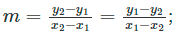where x1 ≠ x2

2.      An acute angle θ between lines L1 and L2 with slopes m1 and m2 is given by1 + m1.m2 ≠ 0.

3.      Equation of the line passing through the points (x1, y1) and (x2, y2) is given by:4.      Equation of the line making a and b intercepts on the x- and y-axis respectively is: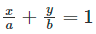5.      The perpendicular distance d of a line Ax + By + C = 0 from a point (x1, y1) is: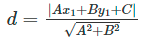6.      The distance between the two parallel lines Ax + By + C1 and Ax + By + C2 is given by: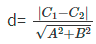### Maths Formulas for Class 11: Chapter 11 Conic Sections

A circle is a geometrical figure where all the points in a plane are located equidistant from the fixed point on a given plane.

1.      The equation of the circle with the centre point (h, k) and radius r is given by (xh)2 + (yk)2 = r2

2.      The equation of the parabola having focus at (a, 0) where a > 0 and directrix x = –a is given by: y2 = 4ax

### Maths Formulas for Class 11: Chapter 12 Introduction to Three Dimensional Geometry

The three planes determined by the pair of axes are known as coordinate planes with XY, YZ and ZX planes.

### Maths Formulas for Class 11: Chapter 13 Limits and Derivatives

A limit of a function at a certain point holds a common value of the left as well as the right hand limits, if they coincide with each other.

1.      For functions f and g, the following property holds true:

3.      The derivative of a function f at a holds as: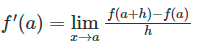4.      The derivative of a function f at a given point x holds as:5.      For the functions u and v, the following holds true:

6.      Standard derivatives:

### Maths Formulas for Class 11: Chapter 14 Mathematical Reasoning

1. Statements: A statement is a sentence which is either true or false, but not both simultaneously.

For example: "A triangle has four sides.", "New Delhi is the capital of India." are the statements.

2. Negation of a statement: Negation of a statement p: If p denotes a statement, then the negation of p is denoted by p.

3. Compound statement: A statement is a compound statement, if it is made up of two or more smaller statements. The smaller statements are called component statements of the compound statement.

The compound statements are made by:
(i) Connectives: "AND", "OR"
(ii) Quantifiers: "There exists", "For every"
(iii) Implications: The meaning of implications “If ”, “only if ”, “ if and only if ”.

(a) "p  q" : p is sufficient condition for q or p implies q.
q is necessary condition for p.
The converse of a statement p
q is the statement q p.
p
q together with its converse, gives p if and only if q.

(b) "p  q"
A sentence with if p, then q can be written in the following ways.

• p implies q (denoted by p q)
• p is a sufficient condition for q
• q is a necessary condition for p
• p only if q
• q implies p

4. Contrapositive: The contrapositive of a statement p q is the statement q ⇒∼p.

New statements, Special words/phrases

5. Contradiction: If to check whether p is true we assume negation p is true.

6. Validating statements: Checking of a statement whether it is true or false. The validity of a statement depends upon which of the special.

The following methods are used to check the validity of statements:

(i) direct method

(ii) contrapositive method

(iv) using a counter example.

### Maths Formulas for Class 11: Chapter 15 Statistics

1. Mean Deviation for the ungrouped data:

2. Mean Deviation for the grouped data:3. Variance and Standard Deviation for the ungrouped data:4. Variance and Standard Deviation of a frequency distribution (discrete):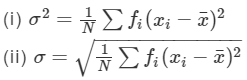5. Variance and Standard Deviation of a frequency distribution (continuous):### Maths Formulas for Class 11: Chapter 16 Probability

1.      Coin: On tossing a coin there are two possibilities, either head may come up or tail may come up.

2.      Die: A die is a well-balanced cube with its six faces marked with numbers (dots) from 1 to 6, one number on the one face. The plural of a die is dice.

3.      Cards: A pack of cards consists of four suits, i.e., Spades, Hearts, Diamonds and Clubs. Each suit consists of 13 cards, nine cards numbered 2, 3, 4, ......, 10 and an Ace, a King, a Queen and a Jack or Knave. Colour of Spades and Clubs is black and that of Hearts and Diamonds is red. Ace, King, Queen and Jack cards are called Face cards.

4.      Random Experiment: An experiment, whose outcomes cannot be predicted with certainty in advance is called a random experiment. For example, on tossing a coin, we cannot predict whether head will come up or tail will come up.

5.      Event: Every subset of a sample space is called an event.

6.      Types of Events:

• Simple Event: Single element of the sample space is called a simple event. It is denoted by S.
• Compound Event: Compound event is the joint occurrence of two or more events.
• Sure Event: In a sure event, a set of all the favourable outcomes is the sample event itself. Its probability is always 1.
• Impossible Event: If E is an impossible event, then S ∩ E = ϕ and the probability of impossible event is 0.
• Equally Likely Events: Two events are said to be equally likely, if none of them is expected to occur in preference to the other. For example, if we toss a coin, each outcome head or tail is equally likely to occur.
• Mutually Exclusive Event: Two events E1 and E2 are said to be mutually exclusive if E1  E2 = ϕ. On tossing a coin two events are possible, (i) coming up a head excludes coming of a tail, (ii) coming up a tail excludes coming of a head. Coming of a head and coming of a tail are mutually exclusive events.
• Independent Events: Occurrence of one event does not depend on the occurrence of other. For example, on tossing two coins simultaneously occurrence of one toss does not depend upon the occurrence of the second one.
• Exhaustive Events: Exhaustive events consist of all possible outcomes.
• Complement of an Event: The complement of an event E with respect to the sample space S is the set of all elements of S, which are not in E. The compliment of E is denoted by E'.